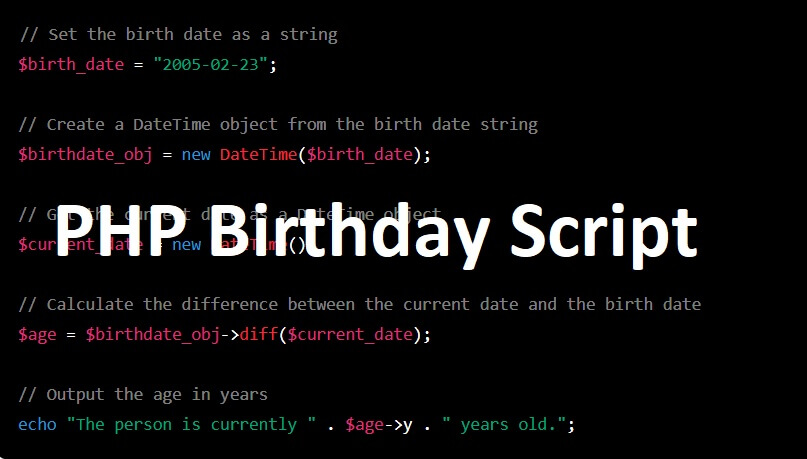# Get Current and Next Birthday Using PHP Script of Any PersonLearn how to use PHP Script to calculate a person’s current age based on their birth date, and also how to determine their next birthday. This tutorial will provide you with the code you need to easily generate a simple solution for getting the current and next birthday of any person.

To get the current age of a person based on their birth date in PHP Script, you can use the DateTime class and the diff() method to calculate the difference between the birth date and the current date. Here’s an example:## PHP Code for Current Birthday

```// Set the birth date as a string
\$birth_date = "2005-02-23";

// Create a DateTime object from the birth date string
\$birthdate_obj = new DateTime(\$birth_date);

// Get the current date as a DateTime object
\$current_date = new DateTime();

// Calculate the difference between the current date and the birth date
\$age = \$birthdate_obj->diff(\$current_date);

// Output the age in years
echo "The person is currently " . \$age->y . " years old.";
```

This code will output the current age of the person based on the birth date provided. For example, if the current date is 2023-02-25, the output would be:

The person is currently 18 years old.

## Method 2

```\$dob = "1999-02-28";
\$currentAge = floor((time() - strtotime(\$dob)) / 31556926);
echo "\$currentAge";```

If you want to get the person’s next birthday, you can use the modify() method of the DateTime object to set the year to the current year, and then add one year if the current date is after the person’s birthday. Here’s an example:

## PHP Code for Next Upcoming Birthday

```// Set the birth date as a string
\$birth_date = "2005-02-23";

// Create a DateTime object from the birth date string
\$birthdate_obj = new DateTime(\$birth_date);

// Set the year of the birth date to the current year
\$birthdate_obj->modify(date('Y') . '-' . \$birthdate_obj->format('m-d'));

// If the current date is after the person's birthday, add one year
if (\$birthdate_obj < \$current_date) {
\$birthdate_obj->modify('+1 year');
}

// Calculate the difference between the current date and the next birthday
\$age = \$current_date->diff(\$birthdate_obj);

// Output the next birthday
echo "The person's next birthday is on " . \$birthdate_obj->format('Y-m-d') . ", which is in " . \$age->days . " days.";

```

This code will output the person’s next birthday and the number of days until their next birthday. For example, if the current date is 2023-02-25, the output would be:

The person’s next birthday is on 2023-02-23, which is in -2 days.

Note that the number of days until the next birthday can be negative if the current date is after the person’s birthday.